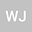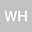Existence of multiple positive solutions for singular p-q-Laplacian problems with critical nonlinearities
••Wei Han
North University of China - South Campus
Author Profile## Abstract

In this article, we consider the following p-q-Laplacian system with singular and critical nonlinearity \begin{equation*} \left \{ \begin{array}{lllll} -\Delta_{p}u-\Delta_{q}u=\frac{h_{1}(x)}{u^{r}}+\lambda\frac{\alpha}{\alpha+\beta}u^{\alpha-1}v^{\beta} \ \ in\ \Omega ,\\ -\Delta_{p}v-\Delta_{q}v=\frac{h_{2}(x)}{v^{r}}+\lambda\frac{\beta}{\alpha+\beta}u^{\alpha}v^{\beta-1} \ \ in\ \Omega, \\ u,v>0 \ \ \ \ \ \ in \ \Omega, \ \ \ \ \ u=v=0 \ \ \ \ \ \ \ on \ \partial\Omega, \end{array} \right. \end{equation*} where Ω is a bounded domain in $\mathbb {R}^{n}$ with smooth boundary $\partial\Omega$. $11,\lambda\in(0,\Lambda_{*})$ is parameter with $\Lambda _{*}$ is a positive constant and $h_{1}(x),h_{2}(x)\in L^{\infty},h_{1}(x),h_{2}(x)>0$. We show the existence and multiplicity of weak solution of equation above for suitable range of $\lambda$.
06 Apr 2021Submitted to Mathematical Methods in the Applied Sciences
08 Apr 2021Submission Checks Completed
08 Apr 2021Assigned to Editor
19 Apr 2021Reviewer(s) Assigned
15 Jul 2021Review(s) Completed, Editorial Evaluation Pending
20 Jul 2021Editorial Decision: Revise Minor